Q&A - Ask Doubts and Get Answers

Sort by :
Clear All
Q
Engineering
306 Views   |

In graphite and diamond, the percentage of p-characters of the hybrid orbitals in hybridisation are respectively :

• Option 1)

33 and 25

• Option 2)

33 and 75

• Option 3)

50 and 75

• Option 4)

67 and 75

As we learned   Graphite - C atom is sp2 hybridised and thermodynamically more stable than diamond at ordinary temperature  - wherein High electrical conductivity is due to presence of delocalised  electron cloud.     Diamond - C atom is sp3 hybridised and has three dimensional network structure - wherein It is bad conductor due to absence of valence electron     Graphite  hybridization  % = ...
Engineering
83 Views   |

Which of the following is a Lewis acid?

• Option 1)

• Option 2)

• Option 3)

NaH

• Option 4)

As we learned   Lewis acids and bases - Lewis defined an acid as a species which accepts electron pair and base which donate an electron. - wherein In lewis acid many acid do not have protons.     It is electron deficient, so it can accept low pair of electron and behave as hewis acid Option 1)       Option 2) Option 3) NaH Option 4)
Engineering
115 Views   |

In the molecular orbital diagram for the molecular ion,  the number of electrons in the  molecular orbital is :

• Option 1)

0

• Option 2)

1

• Option 3)

2

• Option 4)

3

As we learned

Molecular Orbital Theory ( M.O.T ) -

Molecular orbital ( M.O ) Theory was developed by F Hund and  R.S Mulliken in 1932.

-

Number of electrons in

Option 1)

0

Option 2)

1

Option 3)

2

Option 4)

3

View More
Engineering
88 Views   |

For  and the correct order of increasing ionic radii is :

• Option 1)

• Option 2)

• Option 3)

• Option 4)

As we learned   Size of isoelectronic species - Smaller the value of  z/e, larger the size of that species.Smaller z means effective nuclear charge is small hence size is large. -    Isoelectronic Series : When Negative charge increases, the radius of negatively charged ion also increased Option 1)       Option 2) Option 3) Option 4)
Engineering
91 Views   |

An ideal gas undergoes a cyclic process as shown in Figure.Heat absorbed by the system during process CA is :

• Option 1)

• Option 2)

• Option 3)

• Option 4)

As we learned   Isochoric Process - Volume is constant during the process - wherein     Isobaric process - Pressure is constant during the process - wherein      Isobaric  Isochoric  Not Defined               2 - 5  =  -3KJ                     -3 - 5 = -3KJ                         Q+w 8 = Q+3 Q= +5KJ     Option 1)       Option 2) Option 3) Option 4)
Engineering
227 Views   |

Ejection of the photoelectron from metal in the photoelectric effect experiment can be stopped by applying 0.5 V when the radiation of 250 nm is used. The work function of the metal is :

• Option 1)

4 eV

• Option 2)

4.5 eV

• Option 3)

5 eV

• Option 4)

5.5 eV

As we learned    Photoelectric Effect - - wherein where m is the mass of the electron u is the velocity associated with the ejected electron. h is plank’s constant. v is frequency of photon, v0 is threshold frequency of metal.   ltopping potential = 0.5 eV   Option 1) 4 eV Option 2) 4.5 eV Option 3) 5 eV Option 4) 5.5 eV
Engineering
213 Views   |

Which of the following statements about colloids is False ?

• Option 1)

Freezing point of colloidal solution is lower than true solution at same concentration of a solute.

• Option 2)

Colloidal particles can pass through ordinary filter paper.

• Option 3)

When silver nitrate solution is added to potassium iodide solution, a negatively charged colloidal solution is formed.

• Option 4)

When excess of electrolyte is added to colloidal solution, colloidal particle will be precipitated.

As we learned   Properties of Colloidal Solutions - Colligative Properties - wherein Colloidal particles being bigger aggregates, the number of particles in a colloidal solution is comparatively small as compared to a true solution. Hence, the value of colligative properties (osmotic pressure, lowering in vapour, pressure, depression in freezing point, etc.) are of small order compared to true...
Engineering
106 Views   |

For which of the following reactions,  is equal to  ?

• Option 1)

• Option 2)

• Option 3)

• Option 4)

As we learned Molar heat capacity for isobaric process C(p) -   - wherein or     Therefore ;  Option 1)       Option 2) Option 3) Option 4)
Engineering
98 Views   |

decomposes to  and  and follows first order kinetics. After 50 minutes, the pressure inside the vessel increases from 50 mmHg to 87.5 mmHg.
The pressure of the gaseous mixture after 100 minute at constant temperature will be :

• Option 1)

175.0 mmHg

• Option 2)

116.25 mmHg

• Option 3)

136.25 mmHg

• Option 4)

106.25 mmHg

As we learned   First Order Reaction - The rate of the reaction is proportional to the first power of the concentration of the reactant. - wherein Formula: R            P a                 0 a-x             x   [differentiate rate law] Unit of         t=0                50                    0                    0 t=50min                                                ...
Engineering
1473 Views   |

When an electric current is passed through acidified water, 112 mL of hydrogen gas at N.T.P. was collected at the cathode in 965 seconds. The current assed, inampere , is :

• Option 1)

1.0

• Option 2)

0.5

• Option 3)

0.1

• Option 4)

2.0

As we learned   Faraday's first law of electrolysis - The mass of any substance deposited or dissolved at any electrode during electrolysis is directly propotional to the quantity of electricity passed through the solution. - wherein W = Z I t Z = mass of electrolyte get deposited Z = electro chemical equivalent   j = 1 qupere   Option 1) 1.0 Option 2) 0.5 Option 3) 0.1 Option 4) 2.0
Engineering
110 Views   |

In which of the following reactions, an increase in the volume of the container will favour the formation of products ?

• Option 1)

• Option 2)

• Option 3)

• Option 4)

As we learned   General expression of solubility product - - wherein Its solubility product is       Volume Increases, Pressure decreases, reaction proceed in whitch direction where number of gaseous moles increases.   Option 1)       Option 2) Option 3) Option 4)
Engineering
109 Views   |

The minimum volume of water required to dissolve 0.1 g lead(II) chloride to get a saturated solution(  is :

• Option 1)

0.36 L

• Option 2)

17.98 L

• Option 3)

0.18 L

• Option 4)

1.798 L

As we learned                                     2s Option 1) 0.36 L Option 2) 17.98 L Option 3) 0.18 L Option 4) 1.798 L
Engineering
116 Views   |

A sample of  is converted by heat to NaCl with a loss of 0.16 g of oxygen. The residue is dissolved in water and precipitated as AgCl. The mass of AgCl (in g) obtained will be : (Given : Molar mass of )

• Option 1)

0.35

• Option 2)

0.41

• Option 3)

0.48

• Option 4)

0.54

As we learned                                                on CI the weight of   =0.48g     Option 1) 0.35   Option 2) 0.41 Option 3) 0.48 Option 4) 0.54
Engineering
122 Views   |

Total number of lone pair of electrons in     ion is :

• Option 1)

12

• Option 2)

3

• Option 3)

6

• Option 4)

9

As we learn

Lewis structure -

1. pair of bonded electrons is by means of a dash (-) usually called a bond

2. Lone pairs or non-bonded electrons are represented by dots

3. Electron represent in the last shell of atoms are called valence electrons.

- wherein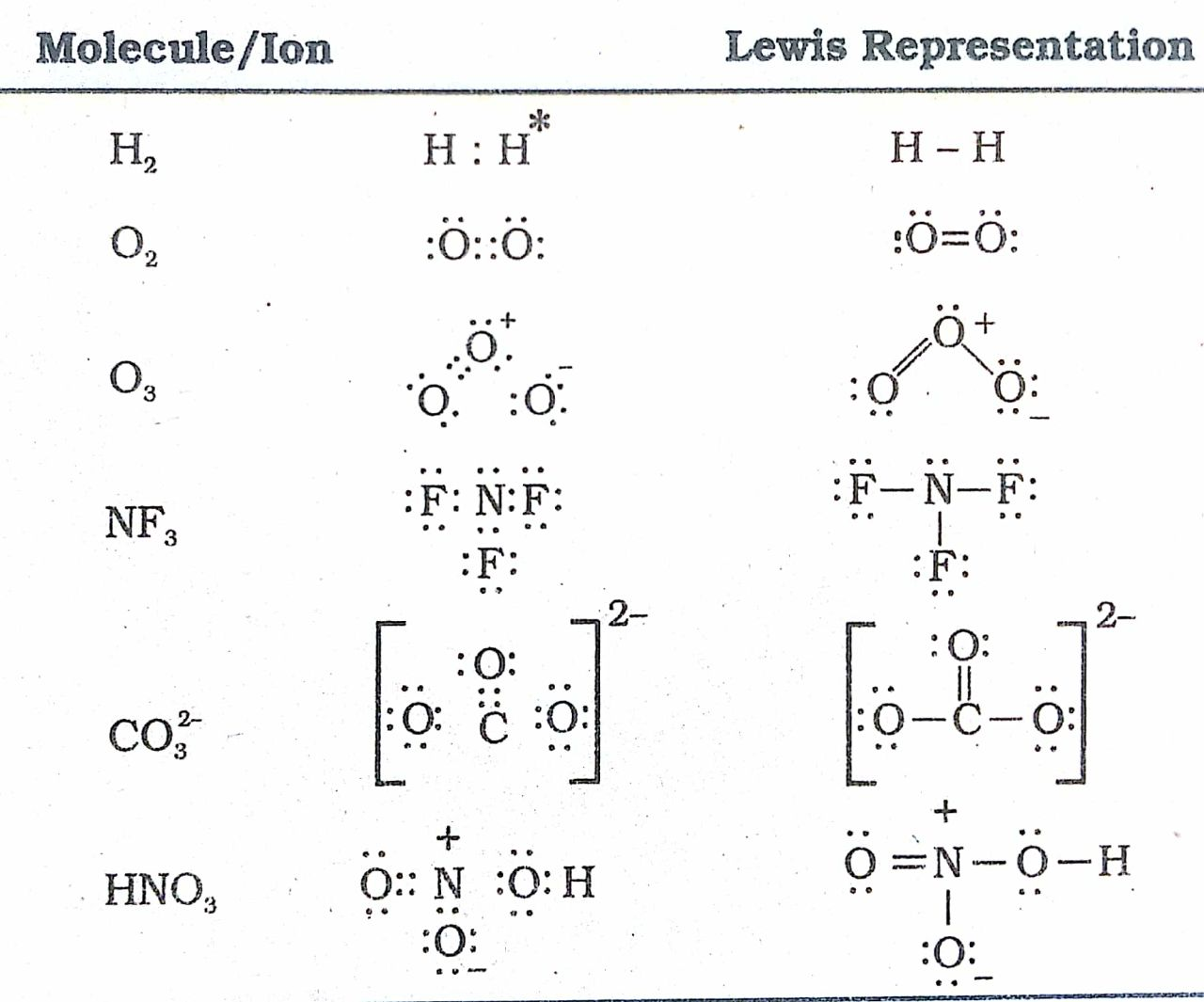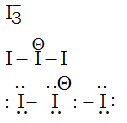No. of lone pairs =9

Option 1)

12

Option 2)

3

Option 3)

6

Option 4)

9

View More
Engineering
133 Views   |

Which of the following salts is the most basic in aqueous solution ?

• Option 1)

• Option 2)

• Option 3)

• Option 4)

As we learned

Conjugate acid base pair -

The acid base pair that differ only by one proton is called a conjugate acid base pair.

-

Salt of weak acid and strong base is basic in solution due to hydrolysis

Option 1)

Option 2)

Option 3)

Option 4)

View More
Engineering
186 Views   |

The recommended concentration of fluoride ion in drinking water is up to 1 ppm as fluoride ion is required to make
teeth enamel harder by converting [3Ca3(PO4)2.Ca(OH)2] to :

• Option 1)

[3{Ca(OH)2}.CaF2]

• Option 2)

[CaF2]

• Option 3)

[3(CaF2).Ca(OH)2]

• Option 4)

[3Ca3(PO4)2.CaF2]

As we learned Food preservatives - Prevent spoilage of food due to microbial growth - wherein Eg: Sodium benzoate (), sugar, table salt, salts of sorbic acid and propionic acids    Soluble fluoride is often added to drinking water to bring its concentration upto 1ppm. The  ions make the enamel on the teeth much harder by converting hydroxyl apatite  the enamel on the surface of the teeth, into...
Engineering
145 Views   |

The oxidation states of Cr in [Cr(H2O)6]Cl3, [Cr(C6H6)2], and K2[Cr(CN)2(O)2(O2)(NH3)] respectively are :

• Option 1)

+3, 0, and +4

• Option 2)

+3, +4, and +6

• Option 3)

+3, +2, and +4

• Option 4)

+3, 0, and +6

As we learned Oxidation number of central metal atom - The charge the central atom would carry if all the ligands are removed along with the electron pairs that are shared with the central atom. -     Option 1) +3, 0, and +4 Option 2) +3, +4, and +6 Option 3) +3, +2, and +4 Option 4) +3, 0, and +6
Engineering
605 Views   |

An aqueous solution contains 0.10 M H2S and 0.20 M HCl. If the equilibrium constants for the formation of HS from
H2S is 1.0×10−7 and that of S2− from HS ions is 1.2×10−13 then the concentration of S2− ions in aqueous solution is :

• Option 1)

• Option 2)

• Option 3)

• Option 4)

As we lerned Ionization reaction of dibasic acid - - wherein    are called first and second ionization constant.     t = 0        0.1          0.2 t = eq    0.1-x         0.2+2x    x    (x<<0.1)   Option 1) Option 2) Option 3) Option 4)
Engineering
97 Views   |

The compound that does not produce nitrogen gas by the thermal decomposition is :

• Option 1)

• Option 2)

• Option 3)

• Option 4)

As we learned Laboratory method of nitrogen preparation - By heating an aqueous solution of NH4Cl and NaNO2 - wherein       Option 1) Option 2) Option 3) Option 4)
Engineering
90 Views   |

The major product of the following reaction is :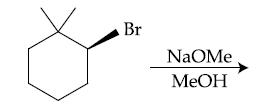• Option 1)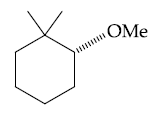• Option 2)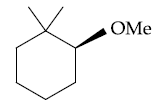• Option 3)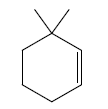• Option 4)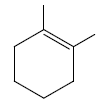As we learned Alcohol formation by hydration of alkenes - Follow electrophilic addition mechanism (Markownikoff) - wherein     Option 1) Option 2) Option 3) Option 4)
Exams
Articles
Questions